Display Accessibility Tools

##Shapes and Designs: Two-Dimensional Geometry

### Properties of Polygons: Understand the properties of polygons that affect their shape.

• Explore the ways that polygons are sorted into families according to the number and length of their sides and the size of their angles
• Explore the patterns among interior and exterior angles of a polygon
• Explore the patterns among side lengths in a polygon
• Investigate the symmetries of a shape-rotation or reflection
• Determine which polygons fit together to cover a flat surface and why
• Reason about and solve problems involving various polygons

### Relationships Among Angles: Understand special relationships among angles.

• Investigate techniques for estimating and measuring angles
• Use tools to sketch angles
• Reason about the properties of angles formed by parallel lines and transversals
• Use information about supplementary, complementary, vertical, and adjacent angles in a shape to solve for an unknown angle in a multi-step problem

### Constructing Polygons: Understand the properties needed to construct polygons.

• Draw or sketch polygons with given conditions by using various tools and techniques such as freehand, use of a ruler and protractor, and use of technology
• Determine what conditions will produce a unique polygon, more than one polygon, or no polygon, particularly triangles and quadrilaterals
• Recognize the special properties of polygons such as angle sum, side-length relationships and symmetry, that make them useful in building, design, and nature
• Solve problems that involve properties of shapes

##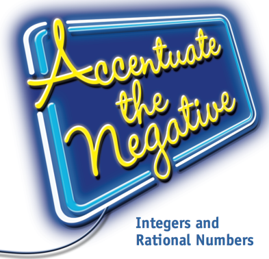Accentuate the Negative: Integers and Rational Numbers

### Rational Numbers: Develop understanding of rational numbers by including negative rational numbers.

• Explore relationships between positive and negative numbers by modeling them on a number line
• Use appropriate notation to indicate positive and negative numbers
• Compare and order positive and negative rational numbers (integers, fractions, decimals, and zero) and locate them on a number line
• Recognize and use the relationship between a number and its opposite (additive inverse) to solve problems
• Relate direction and distance to the number line
• Use models and rational numbers to represent and solve problems

### Operations With Rational Numbers: Develop understanding of operations with rational numbers and their properties.

• Develop and use different models (number line, chip model) for representing addition, subtraction, multiplication, and division
• Develop algorithms for adding, subtracting, multiplying, and dividing integers
• Interpret and write mathematical sentences to show relationships and solve problems
• Write and use related fact families for addition/subtraction and multiplication/division to solve simple equations
• Use parentheses and the Order of Operations in computations
• Understand and use the Commutative Property for addition and multiplication
• Apply the Distributive Property to simplify expressions and solve problems

##Stretching and Shrinking: Understanding Similarity

### Similar Figures: Understand what it means for figures to be similar.

• Identify similar figures by comparing corresponding sides and angles
• Use scale factors and ratios to describe relationships among the side lengths, perimeters, and areas of similar figures
• Generalize properties of similar figures
• Recognize the role multiplication plays in similarity relationships
• Recognize the relationship between scale factor and ratio in similar figures
• Use informal methods, scale factors, and geometric tools to construct similar figures (scale drawings)
• Compare similar figures with nonsimilar figures
• Distinguish algebraic rules that produce similar figures from those that produce nonsimilar figures
• Use algebraic rules to produce similar figures
• Recognize when a rule shrinks or enlarges a figure
• Explore the effect on the image of a figure if a number is added to the x- or y-coordinates of the figure’s vertices

### Reasoning With Similar Figures Develop strategies for using similar figures to solve problems.

• Use the properties of similarity to find distances and heights that cannot be measured directly
• Predict the ways that stretching or shrinking a figure will affect side lengths, angle measures, perimeters, and areas
• Use scale factors or ratios to find missing side lengths in a pair of similar figures
• Use similarity to solve real-world problems

##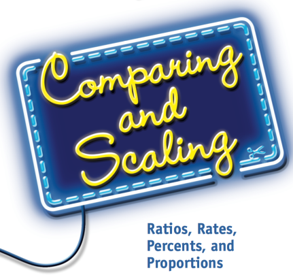Comparing and Scaling: Ratios, Rates, Percents, and Proportions

### Ratios, Rates, and Percents: Understand ratios, rates, and percents.

• Use ratios, rates, fractions, and percents to write statements comparing two quantities in a given situation
• Distinguish between and use both part-to-part and part-to-whole ratios in comparisons
• Use percents to express ratios and proportions
• Recognize that a rate is a special ratio that compares two measurements with different units
• Analyze comparison statements made about quantitative data for correctness and quality
• Make judgments about which kind of comparison statements are most informative or best reflect a particular point of view in a specific situation

### Proportionality: Understand proportionality in tables, graphs, and equations.

• Recognize that constant growth in a table, graph, or equation is related to proportional situations
• Write an equation to represent the pattern in a table or graph of proportionally related variables
• Relate the unit rate and constant of proportionality to an equation, graph, or table describing a proportional situation

### Reasoning Proportionally: Develop and use strategies for solving problems that require proportional reasoning.

• Recognize situations in which proportional reasoning is appropriate to solve the problem
• Scale a ratio, rate, percent, or fraction to make a comparison or find an equivalent representation
• Use various strategies to solve for an unknown in a proportion, including scaling, rate tables, percent bars, unit rates, and equivalent ratios
• Set up and solve proportions that arise from real-world applications, such as finding discounts and markups and converting measurement units

##Moving Straight Ahead: Linear Relationships

### Linear Relationships: Recognize problem situations in which two or more variables have a linear relationship to each other.

• Identify and describe the patterns of change between the independent and dependent variables for linear relationships represented by tables, graphs, equations, or contextual settings
• Construct tables, graphs, and symbolic equations that represent linear relationships
• Identify the rate of change between two variables and the x- and y-intercepts from graphs, tables, and equations that represent linear relationships
• Translate information about linear relationships given in a context, a table, a graph, or an equation to one of the other forms
• Write equations that represent linear relationships given specific pieces of information, and describe what information the variables and numbers represent
• Make a connection between slope as a ratio of vertical distance to horizontal distance between two points on a line and the rate of change between two variables that have a linear relationship
• Recognize that y = mx represents a proportional relationship
• Solve problems and make decisions about linear relationships using information given in tables, graphs, and equations

### Equivalence: Understand that the equality sign indicates that two expressions are equivalent.

• Recognize that the equation y = mx + b represents a linear relationship and means that mx + b is an expression equivalent to y
• Recognize that linear equations in one unknown, k = mx + b or y = m(t) + b, where k, t, m, and b are constant numbers, are special cases of the equation y = mx + b
• Recognize that finding the missing value of one of the variables in a linear relationship,y = mx + b, is the same as finding a missing coordinate of a point (x, y) that lies on the graph of the relationship
• Solve linear equations in one variable using symbolic methods, tables, and graphs
• Recognize that a linear inequality in one unknown is associated with a linear equation
• Solve linear inequalities using graphs or algebraic reasoning
• Solve linear inequalities using graphs or algebraic reasoning
• Write and interpret equivalent expressions

##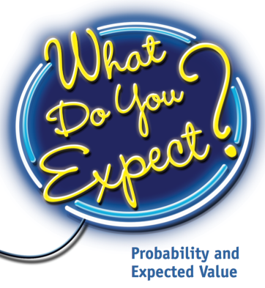What Do You Expect?: Probability and Expected Value

### Experimental and Theoretical Probabilities: Explore and learn basic probability concepts and understand that you can build probability models by gathering data from experiments (experimental probability) and by analyzing the possible equally likely outcomes (theoretical probability).

• Recognize that probabilities are useful for predicting what will happen over the long run
• For an event described in everyday language, identify the outcomes in the sample space, which compose the event
• Interpret experimental and theoretical probabilities and the relationship between them and recognize that experimental probabilities are better estimates of theoretical probabilities when they are based on larger numbers
• Distinguish between equally likely and non-equally likely outcomes by collecting data and analyzing experimental probabilities
• Realize that the probability of simple events is the fraction of outcomes in the sample space for which the event occurs
• Recognize that the probability of a chance event is a number between 0 and 1 that expresses the likelihood of the event occurring
• Approximate the probability of a chance event by collecting data on the chance process that produces it and observing its long-run relative frequency, and predict the approximate relative frequency given the probability
• Determine the fairness of a game

### Reasoning with Probability: Explore and develop probability models by identifying possible outcomes and analyze probabilities to solve problems.

• Develop a uniform probability model by assigning equal probability to all outcomes, and use the model to determine probabilities of events
• Develop a probability model (which may not be uniform) by observing frequencies in data generated from a chance process
• Represent sample spaces for simple and compound events and find probabilities using organized lists, tables, tree diagrams, area models, and simulation
• Realize that, just as with simple events, the probability of a compound event is the fraction of outcomes in the sample space for which the compound event occurs
• Design and use a simulation to generate frequencies for simple and compound events
• Analyze situations that involve two stages (or two actions)
• Use area models to analyze the theoretical probabilities for two-stage outcomes
• Analyze situations that involve binomial outcomes
• Use probability to calculate the long-term average of a game of chance
• Determine the expected value of a probability situation
• Use probability and expected value to make a decision

##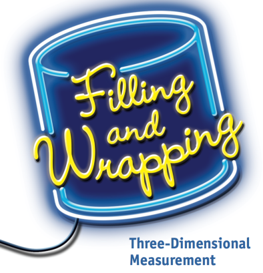Filling and Wrapping: Three Dimensional Measurement

### Surface Area and Volume of Polygonal Prisms and Cylinders: Understand surface area and volume of prisms and cylinders and how they are related.

• Describe prisms by using their vertices, faces, and edges
• Visualize three-dimensional shapes and the effects of slicing those shapes by planes
• Deepen understanding of volume and surface area of rectangular prisms
• Estimate and calculate surface area and volume of polygonal prisms by relating them to rectangular prisms
• Explore the relationships between the surface area and volume of prisms
• Relate surface area and volume for common figures, especially optimization of surface area for fixed volume
• Predict the effects of scaling dimensions on linear, area, and volume measures of cylinders and solid figures
• Investigate the relationship between volume and surface area of prisms and cylinders
• Use volume and surface area of prisms to develop formulas for volume and surface area of cylinders
• Discover that volumes of prisms and cylinders can be calculated as the product of the height and the area of the base
• Solve problems that involve questions about surface area and volume of solid figures

### Area and Circumference of Circles: Understand the area and circumference of circles and how they are related.

• Relate area of a circle to covering a figure and circumference to surrounding a figure
• Estimate and calculate area and circumference of circles
• Explore the relationship between circle radius (and diameter) and area
• Investigate the connection of π to area calculation by estimating the number of ‘radius squares’ needed to cover a circle
• Investigate the relationship between area and circumference of a circle
• Solve problems that involve questions about area and circumference of circles

### Volume of Spheres, Cones and Pyramids: Understand the relationship between the volume of cylinders to cones, spheres and pyramids.

• Relate volume of cylinders to volume of cones, spheres
• Estimate and calculate volume of spheres, cones and pyramids
• Relate volume of cones to volume of cylinders and volume of pyramids to volume of prisms
• Solve problems that involve questions about surface area and volume of spheres, cones and pyramids

##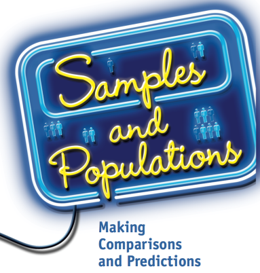Samples and Populations: Making Comparisons and Predictions

### The Process of Statistical Investigation: Understand and use the process of statistical investigation.

• Pose questions, collect and analyze data, and make interpretations to answer questions
• Apply Guidelines for Describing Distributions as a tool to be used with the analyzing and interpreting phases of the statistical investigation process
• Construct and use simple surveys as a method of collecting data

### Analysis of Data Distributions: Understand data distributions and what it means to analyze them.

• Distinguish data and data types
• Recognize data consist of counts or measurements of a variable that are called a distribution of data values
• Distinguish between categorical data and numerical data and identify which graphs and statistics may be used to represent each kind of data
• Use multiple representations
• Organize and represent data using tables, dot plots, line plots, value bar graphs, frequency bar graphs, histograms, and box-and-whisker plots
• Make informed decisions about which graphs/tables are used to display data being analyzed (ties back to questions asked, data types, etc.)
• Recognize that data displayed using a graph shows the overall shape of a distribution and gives a general sense of whether the data values are or are not symmetrical around a central value or if there is something unusual about the shape
• Recognize that a single number may be computed and used to characterize the center or what's typical for a distribution of data
• Distinguish and compute measures of central tendency: the mean, median, or mode of the data
• Identify how the median and mean respond to changes in the number and magnitude of data values in a distribution
• Make informed decisions about which measures of central tendency (mean, median, or mode) may be used to describe a data distribution
• Recognize that variability occurs whenever data are collected and describe the variability in the distribution of a given data set
• Describe the amount of variability in a distribution, noting if the data values are pretty much the same or are quite spread out
• Distinguish and compute measures of spread: range, interquartile range (IQR), and mean absolute deviation (MAD)
• Develop strategies for analyzing and/or comparing data distributions
• Identify which statistical measures of center and spread (mean, median, mode, range, etc.) are most appropriate to use to describe a distribution of data
• Use measures of center and spread to compare data distributions

### Representative Samples: Understand that statistics can be used to gain information about a population by examining a representative sample of the population.

• Use random sampling to select representative samples and use data from these samples to draw conclusions about populations
• Explore the influence of sample size and sample selection processes on measures of center and variability that describe a sample distribution
• Apply concepts from probability to select random samples from populations
• Compare sample distributions using measures of center (mean, median), measures of spread (range, IQR, MAD), and data displays that group data (histograms, box-and-whisker plots)